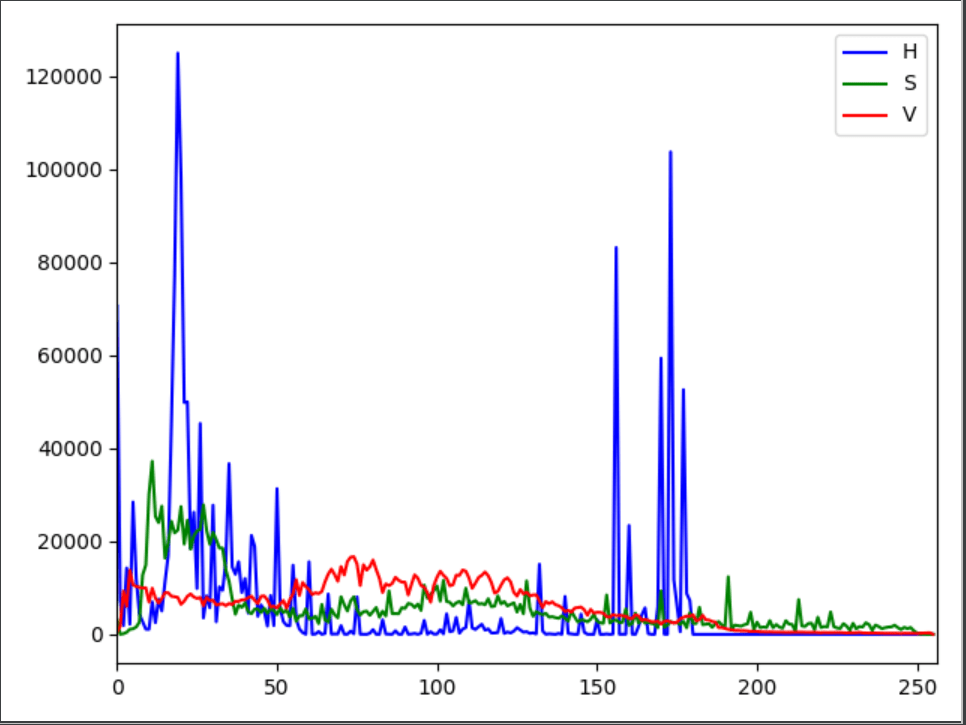# 机器学习图像特征提取—颜色（RGB、HSV、Lab）特征提取并绘制直方图

1 颜色特征

1.1 RGB色彩空间

1.2 HSV色彩空间

1.3 Lab色彩空间

2 使用opencv-python对图像颜色特征提取并绘制直方图

2.1 RGB颜色特征和直方图

2.2 HSV颜色特征和直方图

2.3 Lab颜色特征和直方图

# 1 颜色特征

## 1.1 RGB色彩空间

RGB色彩模式是工业界的一种颜色标准，是通过对红(R)、绿(G)、蓝(B)三个颜色通道的变化以及它们相互之间的叠加来得到各式各样的颜色的，RGB即是代表红、绿、蓝三个通道的颜色，这个标准几乎包括了人类视力所能感知的所有颜色，是运用最广的颜色系统之一。

RGB颜色模型的优点是：

（1）易于理解；

（2）便于硬件实现，现代显示屏一般基于RGB模型；

（3）引入位分辨率(颜色深度)，指一个像素中，每个颜色分量的比特数。位分辨率决定了色彩等级，例如8位颜色深度，每个颜色分量就有256种可能。

RGB颜色模型的缺点在于三个分量均用于表示色调，即如果改变某一个分量的数值，这个像素的颜色就发生了改变。在颜色定位等工程中，使用RGB模型就要同时考虑R、G、B三个变量、较为复杂。

## 1.2 HSV色彩空间

HSV中颜色的参数分别是：色调（H），饱和度（S），明度（V）。对用户来说是一种直观的颜色模型。我们可以从一种纯色彩开始，即指定色彩角H，并让V=S=1，然后我们可以通过向其中加入黑色和白色来得到我们需要的颜色。增加黑色可以减小V而S不变，同样增加白色可以减小S而V不变。

由于HSV是一种比较直观的颜色模型，所以在许多图像编辑工具中应用比较广泛。在 HSV 颜色空间下，比 BGR 更容易跟踪某种颜色的物体，常用于分割指定颜色的物体。

## 1.3 Lab色彩空间

Lab颜色模型是由CIE（国际照明委员会）制定的一种色彩模式。自然界中任何一点色都可以在Lab空间中表达出来，它的色彩空间比RGB空间还要大。另外，这种模式是以数字化方式来描述人的视觉感应， 与设备无关，所以它弥补了RGB和CMYK模式必须依赖于设备色彩特性的不足。

由于Lab的色彩空间要比RGB模式和CMYK模式的色彩空间大。这就意味着RGB以及CMYK所能描述的色彩信息在Lab空间中都能得以影射。Lab颜色模型取坐标Lab，其中L亮度；a的正数代表红色，负端代表绿色；b的正数代表黄色， 负端代表蓝色。

# 2 使用opencv-python对图像颜色特征提取并绘制直方图

``````hist = cv2.calcHist([image],             # 传入图像（列表）
,                 # 使用的通道（使用通道：可选,,）
,               # HistSize
[0.0,255.0])         # 直方图柱的范围
# return->list
``````

## 2.1 RGB颜色特征和直方图

``````import numpy as np
import cv2
import matplotlib.pyplot as plt

# 读取图片
pic_file='./photo/cat.jpg'
cv2.imshow("input",img_bgr)
# 分别获取三个通道的ndarray数据
img_b=img_bgr[:,:,0]
img_g=img_bgr[:,:,1]
img_r=img_bgr[:,:,2]

'''按R、G、B三个通道分别计算颜色直方图'''
b_hist = cv2.calcHist([img_bgr],,None,,[0,255])
g_hist = cv2.calcHist([img_bgr],,None,,[0,255])
r_hist = cv2.calcHist([img_bgr],,None,,[0,255])
m,dev = cv2.meanStdDev(img_bgr)  #计算G、B、R三通道的均值和方差
# img_r_mean=np.mean(r_hist)  #计算R通道的均值
# print(m)
# print(dev)

'''显示三个通道的颜色直方图'''
plt.plot(b_hist,label='B',color='blue')
plt.plot(g_hist,label='G',color='green')
plt.plot(r_hist,label='R',color='red')
plt.legend(loc='best')
plt.xlim([0,256])
plt.show()
cv2.waitKey(0)``````## 2.2 HSV颜色特征和直方图

``````import numpy as np
import cv2
import matplotlib.pyplot as plt

# 读取图片
pic_file='./photo/cat.jpg'
img_hsv = cv2.cvtColor(img_bgr,cv2.COLOR_BGR2HSV)
cv2.namedWindow("input",cv2.WINDOW_GUI_NORMAL)
cv2.imshow("input",img_hsv)

# 分别获取三个通道的ndarray数据
img_h=img_hsv[:,:,0]
img_s=img_hsv[:,:,1]
img_v=img_hsv[:,:,2]

'''按H、S、V三个通道分别计算颜色直方图'''
h_hist = cv2.calcHist([img_hsv],,None,,[0,255])
s_hist = cv2.calcHist([img_hsv],,None,,[0,255])
v_hist = cv2.calcHist([img_hsv],,None,,[0,255])
# m,dev = cv2.meanStdDev(img_hsv)  #计算H、V、S三通道的均值和方差

'''显示三个通道的颜色直方图'''
plt.plot(h_hist,label='H',color='blue')
plt.plot(s_hist,label='S',color='green')
plt.plot(v_hist,label='V',color='red')
plt.legend(loc='best')
plt.xlim([0,256])
plt.show()
cv2.waitKey(0)``````## 2.3 Lab颜色特征和直方图

``````import numpy as np
import cv2
import matplotlib.pyplot as plt

# 读取图片
pic_file='./photo/cat.jpg'
img_lab=cv2.cvtColor(img_bgr,cv2.COLOR_BGR2LAB)
cv2.namedWindow("input",cv2.WINDOW_GUI_NORMAL)
cv2.imshow("input",img_lab)

# 分别获取三个通道的ndarray数据
img_ls=img_lab[:,:,0]
img_as=img_lab[:,:,1]
img_bs=img_lab[:,:,2]

'''按L、A、B三个通道分别计算颜色直方图'''
ls_hist = cv2.calcHist([img_lab],,None,,[0,255])
as_hist = cv2.calcHist([img_lab],,None,,[0,255])
bs_hist = cv2.calcHist([img_lab],,None,,[0,255])
# m,dev = cv2.meanStdDev(img_lab)  #计算L、A、B三通道的均值和方差
# print(m)

'''显示三个通道的颜色直方图'''
plt.plot(ls_hist,label='l',color='blue')
plt.plot(as_hist,label='a',color='green')
plt.plot(bs_hist,label='b',color='red')
plt.legend(loc='best')
plt.xlim([0,256])
plt.show()
cv2.waitKey(0)``````OpenCV—python 颜色空间（RGB，HSV，Lab）与 颜色直方图_SongpingWang的博客-CSDN博客_opencv 颜色